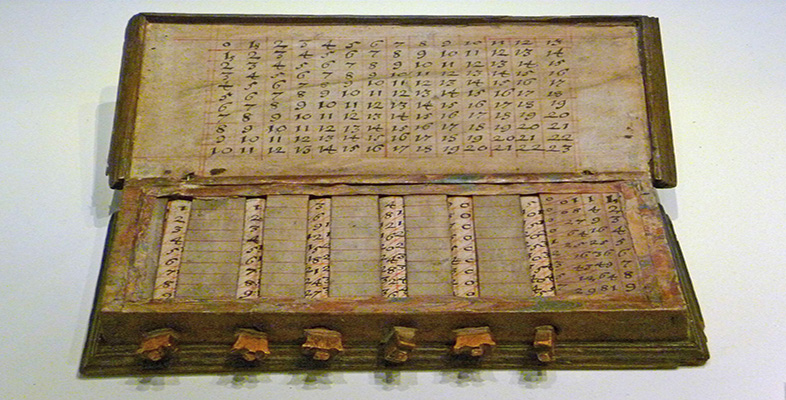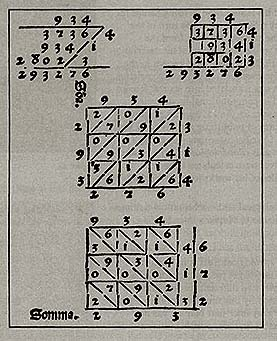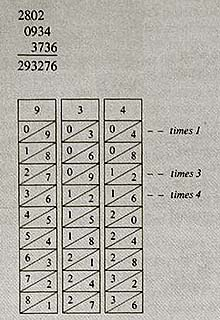John Napier

Start this free course now. Just create an account and sign in. Enrol and complete the course for a free statement of participation or digital badge if available.

Free course

# 1.2 Napier's bones

Before pursuing who logarithms were for (and what they are), we first look briefly at another of Napier's computational aids. For in the years following his death, it was in fact his numerating rods, the so-called Napier's bones, that were more widely known and used. These consisted of the columns of a multiplication table inscribed on rods, which could make the multiplying of two numbers easier by setting down the partial products more swiftly. This simple contrivance was derived from an ancient multiplication method (called ‘lattice’ multiplication – see Box 1), which involved a special layout on paper of the two numbers to be multiplied so as to display the partial products of the multiplication, to facilitate adding them in the right way.

## Box 1: Multiplication on Napier's bones

Consider first various ways of laying out the multiplication of 934 and 314, as given in the Treviso arithmetic of 1478 (the first printed arithmetic book).Note that the result, 293 276, is reached in the middle configuration by adding the figures in the matrix along the diagonal bands, starting from the bottom right (and carrying a digit over to the next band where appropriate).

The same multiplication would be done on Napier's bones by aligning the rods for 9, 3 and 4, and looking horizontally along the times 3, times 1 and times 4 rows to form the partial products to be added, as shown here.MA290_3